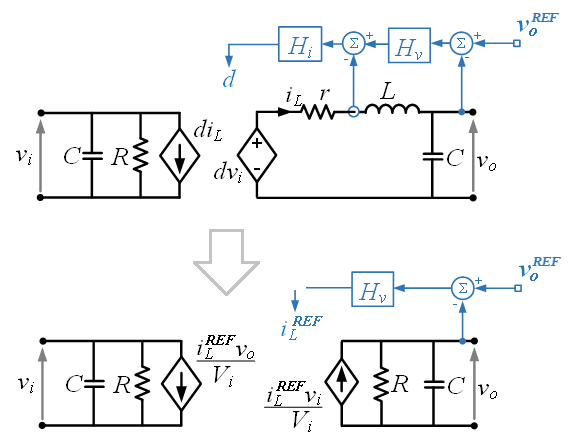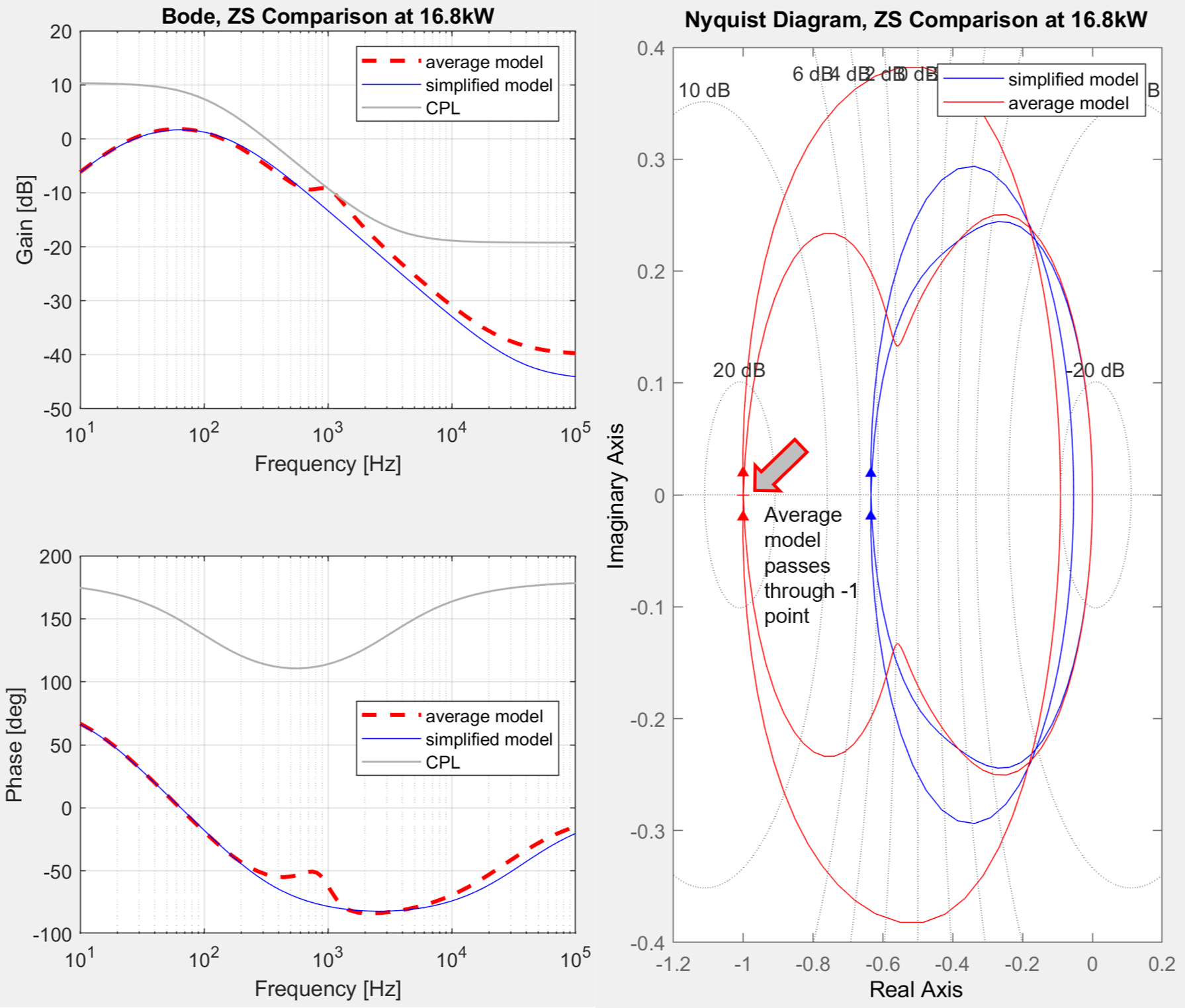LIBRARY

# Reduced Order Modeling of Power Converters for System-level StudiesFig. 1 Simplified model assumes infinite-bandwidth current control.
To support large-scale system-level simulations of distributed point-of-load three-terminal dc and ac power converters, it is desired to develop reduced-order models for improved computational efficiency when higher order response is not a quantity of interest. For a notional 250 kW dc-dc buck converter having switching and current loop bandwidths of 10 kHz and 1 kHz respectively, it is postulated the current loop bandwidth may be treated as infinite and a reduced-order model developed. The order of this simplified model is reduced by not only the order of the omitted current loop controller, but also by a simplification removing the contribution of the output inductor from the model, leading to an ultimate reduction of at least two orders, or more likely three or more.

The challenge lies in analyzing the source-load impedance interactions. If a power converters higher-order source impedance transfer function curve contains salient points not represented in the reduced-order model, there may be cases where the source-load impedance interaction appears stable for a reduced-order model, but is revealed to be unstable by a higher-order average-value model. This may appear in the form of insufficient phase margin where the Bode gain plots intersect, and in the form of Nyquist encirclements of the -1 point. It is desired to determine if a correction can be included in the simplified model.

The simplified model of the characteristic 250 kW dc-dc buck converter was loaded with an identically structured converter representing a constant-power load of 15-30 kW. The source and load transfer functions were then extracted and manipulated to compare load-source impedance interactions for average versus simplified converter models, with a focus on the impact of any salient points in the gain and phase curves which are evident for the higher-order average model, but not for the lower-order simplified model.Fig. 2 Average model unstable while simplified model yet stable.